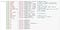What does “PATH math” mean? A lot of us know about STEM (science, technology, engineering, mathematics). PATH is variously decoded, lets say into philosophy, anthropology, theater, history.

A “PATH math” hearkens back to philosophy. “Propositional calculus” as developed by Bertrand Russell and friends, might qualify as a math hatched in the domain of philosophy. As such, professors call it a “logic” and “logic” tends to squeeze in “under” mathematics, thereby providing some kind of “foundation”.

The PATH math I’m talking about has a strong geometrical flavor, tending to arrange a sequence of polyhedrons in volume order, according to various rules for sizing them relative to one another.

Polyhedrons have no set size relative to one another unless that’s specified. For example, a tetrahedron inscribed in a cube as six face diagonals (a cube has six faces) has precisely one third the cube’s volume.

We might show this by filling a tetrahedron of edges two with water, and pouring three of those into a cube with edges of second root of two, thereby filling it precisely.

Here’s a volumes table we’re using:

Some of the terminology is localized and kind of quirky. We also talk about a certain “Jitterbug Transformation” that STEM students likewise know about, although perhaps by a different name.

Figure 5 shows the output of a particular Python program, which is shown below:Fig. 6: volumes in terms of phi-scaled E and S modules

The S and E modules are easy enough to draw or 3D print. We need to scale them, larger and smaller, by the golden mean.

When all linear dimensions of a shape get scaled by a factor, then volume scales by that factor to the third power. That’s why, when we scale the linear dimensions of an S module by phi (the golden mean), we call it S3 (not to be confused with the Synergetics Constant Syn3). Scaled up by phi again, S3 becomes S6 and so on.

When we scale down, multiplying by 1/phi instead, or 0.618…, then volume shrinks by a third power of that linear scale factor. We use a lower case letter s to signifying scaling down (shrinking). s3 = S times (1/phi)(1/phi)(1/phi) and s6 = s3 times (1/phi) to the third power and so on.

How is any of this PATH and not STEM?

The work in which all this geometrical thinking gets introduced is a philosophical work, replete with metaphysical content. A mind versus brain distinction gets developed. Multidisciplinary overview is encouraged.

You will find more writing on Medium regarding this PATH math if you search using the right keywords.

Written by